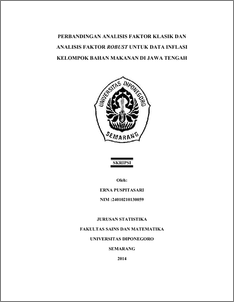# PERBANDINGAN ANALISIS FAKTOR KLASIK DAN ANALISIS FAKTOR ROBUST UNTUK DATA INFLASI KELOMPOK BAHAN MAKANAN DI JAWA TENGAH

PUSPITASARI, ERNA (2014) PERBANDINGAN ANALISIS FAKTOR KLASIK DAN ANALISIS FAKTOR ROBUST UNTUK DATA INFLASI KELOMPOK BAHAN MAKANAN DI JAWA TENGAH. Undergraduate thesis, FSM Undip.Preview
PDF
558Kb

## Abstract

Factor analysis is a statistical method used to describe a set of variables based on common dimensions. Factor analysis that is often used is the classical factor analysis with principal components method. Classical factor analysis can not work properly if the data contained many outliers. In order factor analysis remains optimal in explaining a set of variables even in conditions of data containing many outliers, we need a robust estimator. Through factor analysis is expected to obtain robust high accuracy analysis results for data containing many outliers. Estimator fast-MCD is one of the robust estimator that aims to get the smallest determinant of the covariance matrix. Robust factor analysis with fast-MCD method in this thesis is applied to explain the many subgroups of food at food inflation rate in Central Java into a more modest dimensions. The total proportion of the data variance can be explained by factors that are formed through a robust method of factor analysis in foodstuffs inflation data in Central Java that is equal to 72.9 percent larger than the classical factor analysis method which generates at 53.5 percent. This suggests that a more robust factor analysis method is able to cope with food inflation data in Central Java group containing outliers of the classical factor analysis method. Keywords: Inflation, Outlier, Robust Factor Analysis, fast-MCD.

Item Type: Thesis (Undergraduate) H Social Sciences > HA Statistics Faculty of Science and Mathematics > Department of Statistics 43507 Mr Hasbi Yasin 20 Aug 2014 07:58 20 Aug 2014 07:58

Repository Staff Only: item control page